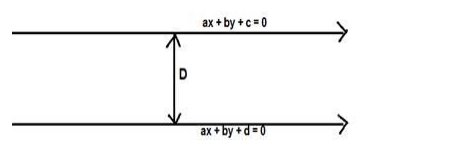# Find the distance between the parallel lines`
Question:

Find the distance between the parallel lines 8x + 15y – 36 = 0 and 8x + 15y + 32 = 0.

Solution:

Given: parallel lines 8x + 15y – 36 = 0 and 8x + 15y + 32 = 0.

To find : distance between the parallel lines

Formula used: The distance between the parallel lines ax $+b y+c=0$ and $a x+b y+d$ $=0$ is,

$D=\frac{|d-c|}{\sqrt{a^{2}+b^{2}}}$Here $a=8, b=15, c=-36, d=32$

$D=\frac{|32-(-36)|}{\sqrt{8^{2}+15^{2}}}=\frac{|32+36|}{\sqrt{64+225}}=\frac{68}{\sqrt{289}}=\frac{68}{17}=4$

The distance between the parallel lines $8 x+15 y-36=0$ and $8 x+15 y+32=0$ is 4 Units